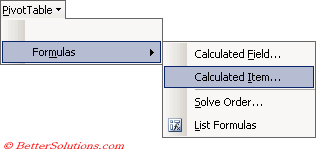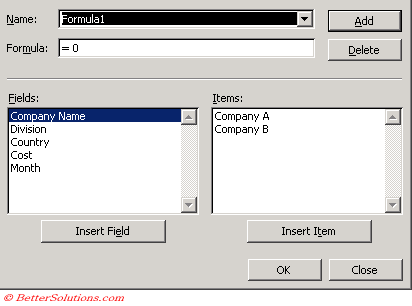# Calculated Items

This is a new row
You can create new items that can appear in your fields that are not part of the original source data.
A calculated field contains user-created formula to determine the value of the item in a particular field.

(PivotTable > Formulas > Calculated Item).You create a calculated item by adding a new item to a field and then defining a formula that calculates the individual values for the item.

### Creating a Calculated Item

Notice that the caption of the dialog box identifies the field you are going to insert the item into.### Removing a Calculated Item

Pivot Table Tools > Options > Formulas > Solve Order
The calculated item solve order dialog box lists all the calculated items in the pivot table report

List Formulas
Options > Formulas > List Formulas